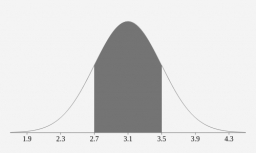# Probability 64174

The banker deals, on average, with five clients a day. Find the probability that the number of clients (in one day) will be greater than 4.

p =  0.1875

### Step-by-step explanation:Did you find an error or inaccuracy? Feel free to write us. Thank you!

Tips for related online calculators
Looking for help with calculating arithmetic mean?
Looking for a statistical calculator?
Looking for a standard deviation calculator?

#### Grade of the word problem:

We encourage you to watch this tutorial video on this math problem: